# 第十四十五周作业

## 要求二

### 题目7-5 矩阵运算

#### 1.实验代码

#include<stdio.h>

int main(void)
{
int a;
int i,j,sum=0,n;
scanf("%d",&n);
for(i=0;i<n;i++){
for(j=0;j<n;j++)
scanf("%d",&a[i][j]);
}
for(i=0;i<n;i++){
for(j=0;j<n;j++) {
if((i+j!=(n-1))&&(i!=(n-1))&&(j!=(n-1)))
sum=sum+a[i][j];}
}
printf("%d",sum);
return 0;
}

#### 2 设计思路

（1）文字描述

（2）流程图#### 3.本题调试过程碰到问题及解决办法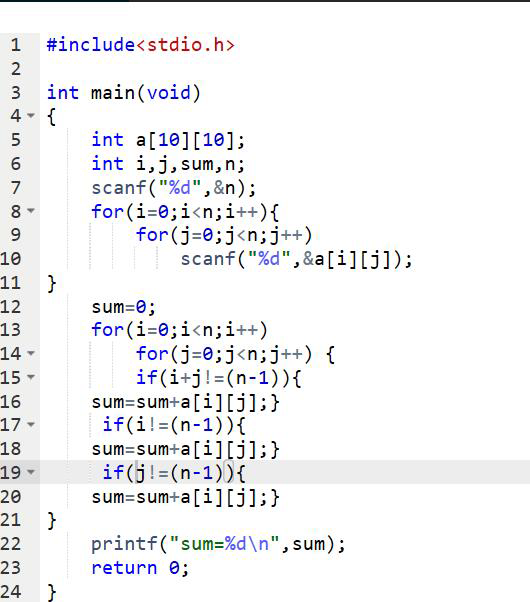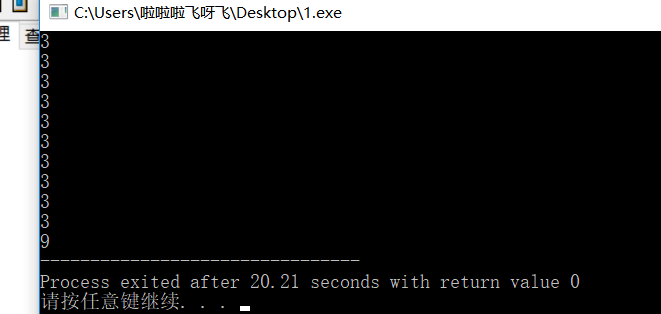4.本题PTA提交列表错误提示解释说明:本题出现了多个错误，编译错误有sum定义了两次，大括号少打了一个，答案错误是由于上面所说的问题导致的。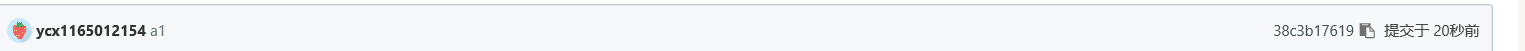要求四

1本周学习了数组，二维数组以及储存多个值并次序输出。

2数组好像一知半解的，还是要多练多问多思考才行。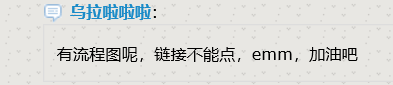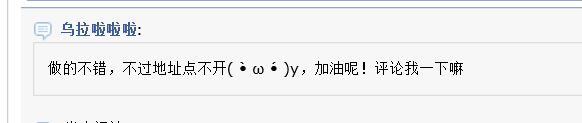12.3 代码行数 100 博客字数 600 总时间 300min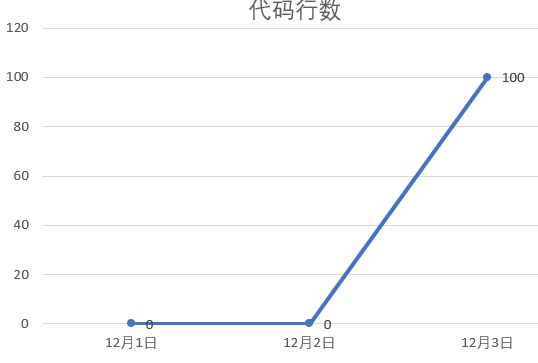posted @ 2017-12-03 21:36  乌拉啦啦啦  阅读(114)  评论(2编辑  收藏  举报